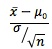### Why should I choose AnalystNotes?

Simply put: AnalystNotes offers the best value and the best product available to help you pass your exams.

##### Subject 7. The Relationship between Confidence Intervals and Tests of Significance
A confidence interval is a range of values within which it is believed that a certain unknown population parameter (often the mean) will fall with a certain degree of confidence. The percentage of confidence is denoted (1 - α)%.

A test of significance is a test to ascertain whether or not the value of an unknown population parameter is as stated by an individual or institution. This test is carried out at a significance level of α, which determines the size of the rejection region.

Note how the confidence interval is related to the test statistic. They are linked by the rejection point(s).

• The confidence interval is:• Recall that the test statistic is:The likelihood that the z-value will be less than the test statistic is what is being tested. Setting up this inequality, and rearranging, the test is:Confidence intervals can be used to test hypotheses. Note that the right side of the equation is the left endpoint of the confidence interval. Essentially, if the confidence interval contains the value of the unknown population parameter as hypothesized under H0, then H0 would not be rejected in a two-sided hypothesis test with corresponding α; if the confidence interval does not contain the value of the unknown population parameter as hypothesized under H0, then H0 would be rejected in a two-sided hypothesis test with corresponding α.

The reason for the above determination is as follows:

• If the hypothesized value does not fall in the confidence interval, then there is a very small chance that the value can be a true value for the unknown parameter, so H0 is almost certainly wrong and will be rejected.
• If the hypothesized value does fall in the confidence interval, then there is a very good chance that the value can be a true value for the unknown parameter, so H0 is definitely possible and will not be rejected.

This comparison can be used only for two-sided tests, not one-sided tests, because confidence intervals cannot be linked with one-sided tests.

Learning Outcome Statements

d. explain a decision rule, the power of a test, and the relation between confidence intervals and hypothesis tests;

CFA® 2022 Level II Curriculum, , Volume 1, Reading 11